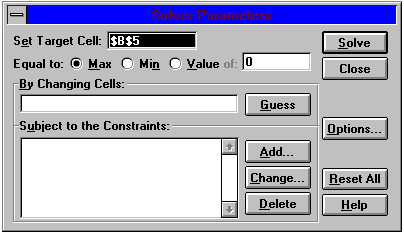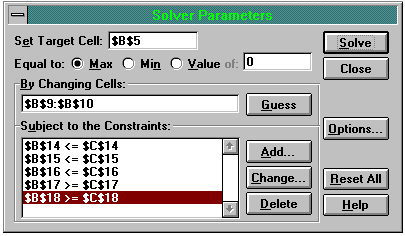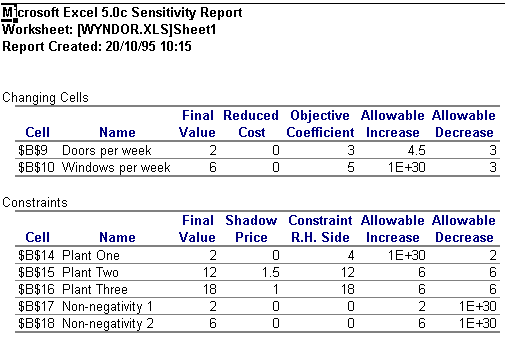Overview

Linear Programming may at first seem basic and easy to do once one gets the hand of it, but in reality it can involve a large variety of different techniques that one can use. Once the basic form is learned, it can be applied to the many different forms that it has and used there as well (such as integer and binary programming being very similar in nature to linear programming). The following will explain the ins and outs of linear programming, as well as some of its similar methodologies.

 Table of Contents

## Graphical Method

In essence, all linear programming consists of is minimizing or maximizing a linear function, which is called the objective of the problem. For example, a problem can consist of minimizing costs, or maximizing profit. This function is subject to a variety of constraints, which are usually represented by equalities / inequalities. This may seem easy at first, but the larger the problem gets, the harder it gets as well. Here is an example, for illustration purposes. 

Find numbers X1 and X2 that maximize the sum X1 + X2 and are subject to the constraints X1 ≥ 0, X2 ≥ 0, and

X1 + 2X2 ≤ 4
4X1 + 2X2 ≤ 12
−X1 + X2 ≤ 1

This problem shows some of the concepts explained above. The problem has two unknowns which the person solving the problem must find. It also has five constraints that the problem solver has to deal with, as they can not break these constraints. The first two are a unique kind of constraint, as they are called non negativity constraints. This type of constraint is found in a majority of linear programming problems, and means that the variables you are solving for can not be less than zero. For example, if you would want to find how many acres of corn or wheat you should plant (the unknown variables), you can not physically plant negative 3 acres, which is why the no negativity constraint exists.

The last three constraints are linear and are inequalities, and care called the main constraints. The beginning of the problem talks about finding the sum that maximize the sum X1 + X2. This is called the objective function of the problem. Linear Programming problems can also involve minimizing this objective function. As there are only two variables, the problem can be solved by graphical means. If there were more than two variables in the objective function, then you could not solve this by graphing, and would have to use another approach. 

Graphing this problem would incur by graphing all of the points that satisfy the constraints. Then, all you have to do is find the point that maximizes (or minimizes in some cases) the objective function. Taking the previous problem into play, you can see it fully graphed in Figure 1. Once all of the constraints are graphed, all one has to do is find the area where all of the inequalities share. This is seen in the grey area below.To solve the problem, we need to find the point (of X1 and X2 that is the maximum of the objective function. This point also needs to follow the constraints, and will be in the grey area from figure 1. The special thing about linear programming problems is that the optimal solution is always on a corner point, so all one needs to do is find the corner point that produces the maximum value for the objective function. This could be done by plugging in all the numbers, but that is a bit much if you have a problem with many constraints. 

The easiest way is to find the slopes of the objective function, and the optimal solution will be at the corner point between the 2 lines that have a slope greater than the objectives slope, and lower than it. This can be done by putting all the slopes on a number line and finding it that way. For example, in this problem the objective function has a slope of -1. Listing all the slopes for the constraints gives us 
-2 -1/2 1

These can be found by taking the coefficient in front of X1, making it negative, then dividing that by the coefficient of X2. As the objective function has a slope of -1, this falls between -2 and -1/2, so the optimal point is between the lines 4X1, + 2X2 = 12 and X1, + 2X2 = 4. Then all one has to do is find the value of X1 and X2, which in this case is 8/3 and 2/3, respectively. Plugging this back into the objective function gives a value of 10/3. This shows that that maximum of this problem and optimal value is 10/3, given the constraints. 

## Solver

Not all problems can be solved by the graphical method. If the objective function has more than 2 variables in it, then one way to solve the problem is through using computer software. There are many types you can find out there, and most have some sort of fee or trial period attached to them. One popular alternative is to use the Excel Solver. This is a add in in Microsoft Excel that comes free as an add on. To enable it, open up Microsoft Excel, go to Tools, Add Ins, and then enable Solver. Then just go to Tools, Solver to open up the add in. 

Before doing any of those though, the spreadsheet has to be set up with the problem. There are a variety of ways to do this, and different set ups just work better for different people. Here is one way to set up the spreadsheet, and this can be modified for just about any linear programming problem; just add more constraints and change all of the variables and such.This setup has the objective function in B5, and it points to the decision variables in B9 and B10. The constraints are listed out in the bottom of the sheet. The B column shows the RHS side of the problem, and it is set up by multiplying it's corresponding C column with the decision variables. Once it is all set up, open up solver and begin plugging in! First fill in the target cell, which will point to the objective function. Then set it to either Max or Min the problem.The changing cells will point to the decision variables as well. For the constraints highlight the C column constraints for the left hand side, set the inequality, and then highlight the B column constraints. You should also go into options and check "Assume Linear Model" if your model is linear. Also check the nonnegativity option. After this is done, click solve and the program will find the optimal values and fill them in for you. Here is what the template should look like before you click solve.Once this is done, you can generate reports to do a more in depth analysis on the results. You can select which one of this three reports you want to generate, or you can select them all. Once you do that, click OK and the reports will be generated into other tabs.These reports can be a great deal of help when you want to make even more decisions about the problem The Answer report gives you more details of the solutions, as well as showing the slack / surplus values of each variable. The sensitivity report shows how sensitivity the solutions are to changes to constraints. Below you can see a print out of the sensitivity report.As you can see, there are quite a few different columns here showing a variety of different information. The final value shows what the final value was for each variable (obviously). The reduced cost shows how much more profitable they would have to be before they would have been optimal. The allowable increase / decrease shows how much the variables can go up / down before they are not optimal anymore. For the constraints, the shadow price is the maximum that the company is willing to pay for one more unit of that constraint. 

Thus concludes the basics of how to solve linear programming problems using solver. Just about any problem can be put into solver and solved within mere seconds once the set up is done, which many people consider to be the hardest part. The only solver would have trouble with are problems that have hundreds of constraints. Then one could just use a more complex software to solve the problem. 

## References

1. Ferguson, Thomas S. <http://www.math.ucla.edu/~tom/LP.pdf>
2. MacDonald, Ziggy. 1995. "Teaching Linear Programming using Microsoft Excel Solver" <http://www.economicsnetwork.ac.uk/cheer/ch9_3/ch9_3p07.htm>
page revision: 19, last edited: 30 Apr 2008 11:46
Unless otherwise stated, the content of this page is licensed under Creative Commons Attribution-ShareAlike 3.0 License